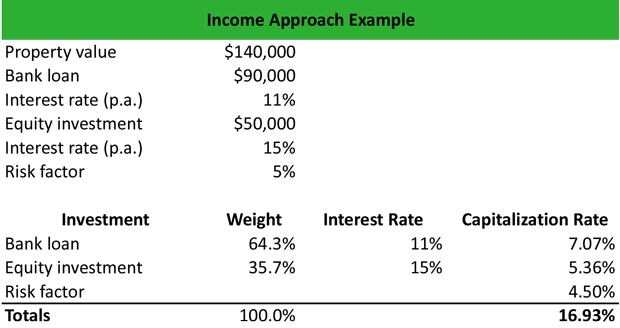# What is Income Approach?

Definition: Income approach is a valuation method used for real estate appraisals that is calculated by dividing the capitalization rate by the net operating income of the rental payments. Investors use this calculation to value properties based on their profitability.

## What Does Income Approach Mean?

What is the definition of income approach? Being one of the most widely implemented valuation methods, the income approach analyzes the expected economic benefits that investors anticipate from a real estate investment. The method discounts the property’s expected cash flows in their present value using the capitalization rate of the property.

The capitalization rate represents the risk of investment and is calculated based on a property’s Interest before Depreciation, Interest, and Taxes (IBDIT) considering the current interest rate on the property’s mortgages, the equity investment in the property, and a risk factor to reflect the real estate investment risks.

Let’s look at an example.

## Example

Nicholas is an investment advisor. He is asked to calculate the capitalization rate of a real estate investment for a client using the income approach valuation method and determine the property’s present value.

Nicholas uses the income statements of the property to calculate the Interest before Depreciation, Interest, and Taxes (IBDIT) at \$85,000. The calculation of the IBDIT includes the costs of building maintenance, the property’s operating expenses, and unusual expenses.

Then, Nicholas calculates the capitalization rate, based on the property value, the interest rate on the bank loan, the return on equity investment, and a risk factor, as follows:The bank loan of \$90,000 represents a weight of 64.3% of the property value. The equity investment of \$50,000 represents a weight of 35.7% of the property value. By multiplying the weight of the bank loan by the annual interest rate and the weight of the equity investment by the expected return on investment, Nicholas calculates the weighted capitalization rates of each weight component. Then, by adding the risk factor of 4.50%, Nicholas calculates the capitalization rate of this investment at 16.93%.

Therefore, the present value of the property is calculated as follows:

Present value of the property = IBDIT / Capitalization rate = \$85,000 / 16.93% = \$502,110

## Summary Definition

Define Income Approach: Income approach is a real estate valuation method used by investors to appraisal a piece of real estate based on its earnings, profitability, and risk.